# Statistics 202 Statistical Aspects of Data Mining Professor

• Slides: 52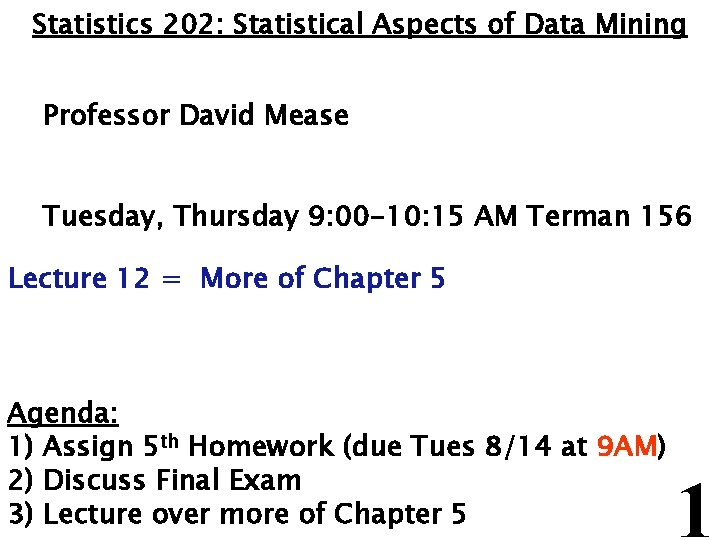Statistics 202: Statistical Aspects of Data Mining Professor David Mease Tuesday, Thursday 9: 00 -10: 15 AM Terman 156 Lecture 12 = More of Chapter 5 Agenda: 1) Assign 5 th Homework (due Tues 8/14 at 9 AM) 2) Discuss Final Exam 3) Lecture over more of Chapter 5 1Homework Assignment: Chapter 5 Homework Part 2 and Chapter 8 Homework is due Tuesday 8/14 at 9 AM. Either email to me ([email protected] edu), bring it to class, or put it under my office door. SCPD students may use email or fax or mail. The assignment is posted at http: //www. stats 202. com/homework. html Important: If using email, please submit only a single file (word or pdf) with your name and chapters in the file name. Also, include your name on the first page. Finally, please put your name and the homework # in the subject of the email. 2Final Exam I have obtained permission to have the final exam from 9 AM to 12 noon on Thursday 8/16 in the classroom (Terman 156) I will assume the same people will take it off campus as with the midterm so please let me know if or 1) You are SCPD and took the midterm on campus but need to take the final off campus 2) You are SCPD and took the midterm off campus but want to take the final on campus More details to come. . . 3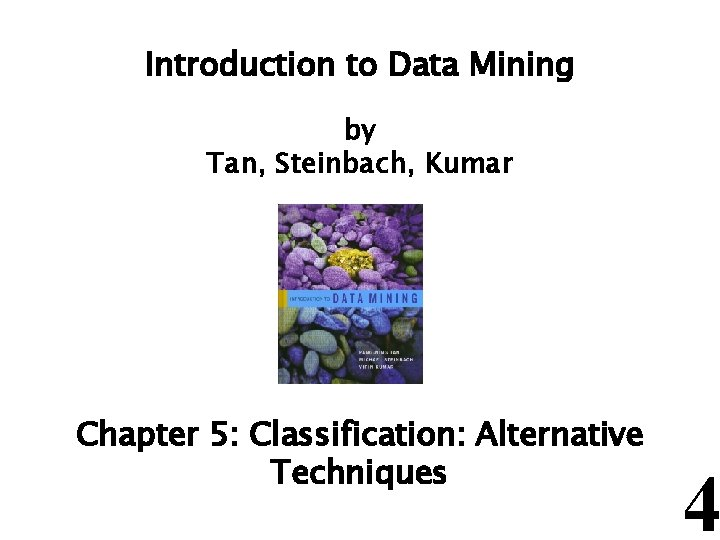Introduction to Data Mining by Tan, Steinbach, Kumar Chapter 5: Classification: Alternative Techniques 4The ROC Curve (Sec 5. 7. 2, p. 298) l ROC stands for Receiver Operating Characteristic Since we can “turn up” or “turn down” the number of observations being classified as the positive class, we can have many different values of true positive rate (TPR) and false positive rate (FPR) for the same classifier. TPR= FPR= l The ROC curve plots TPR on the y-axis and FPR on the x-axis l 5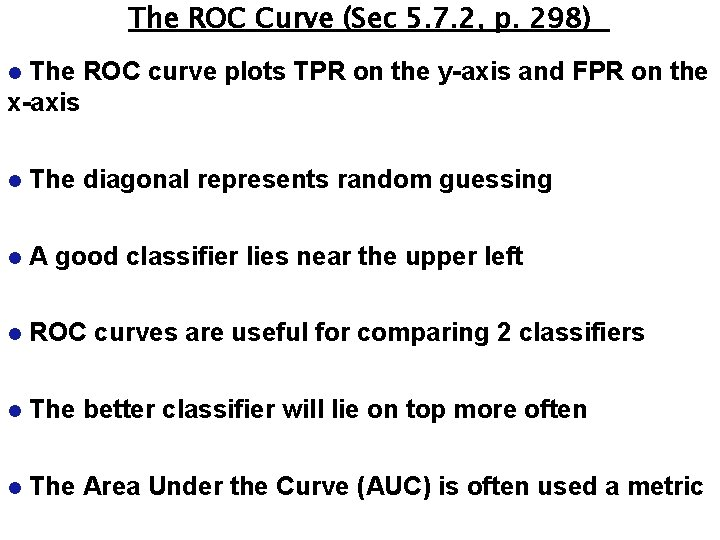The ROC Curve (Sec 5. 7. 2, p. 298) The ROC curve plots TPR on the y-axis and FPR on the x-axis l l The diagonal represents random guessing l A good classifier lies near the upper left l ROC curves are useful for comparing 2 classifiers l The better classifier will lie on top more often l The Area Under the Curve (AUC) is often used a metric 6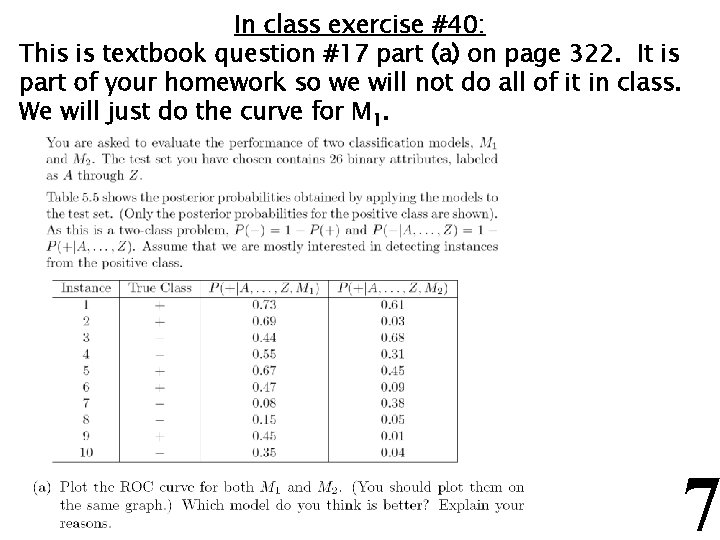In class exercise #40: This is textbook question #17 part (a) on page 322. It is part of your homework so we will not do all of it in class. We will just do the curve for M 1. 7In class exercise #41: This is textbook question #17 part (b) on page 322. 8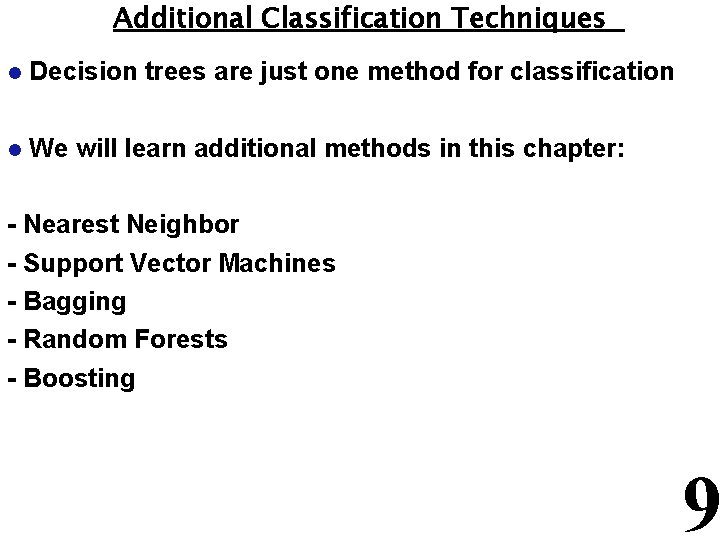Additional Classification Techniques l Decision trees are just one method for classification l We will learn additional methods in this chapter: - Nearest Neighbor - Support Vector Machines - Bagging - Random Forests - Boosting 9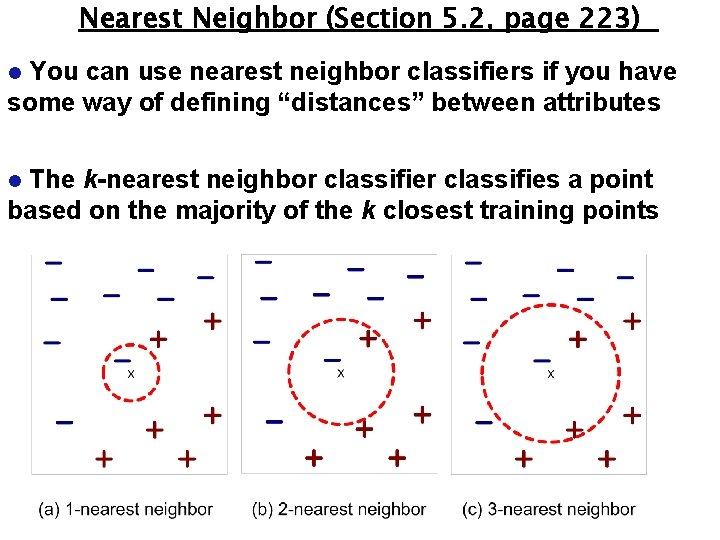Nearest Neighbor (Section 5. 2, page 223) You can use nearest neighbor classifiers if you have some way of defining “distances” between attributes l The k-nearest neighbor classifies a point based on the majority of the k closest training points l 10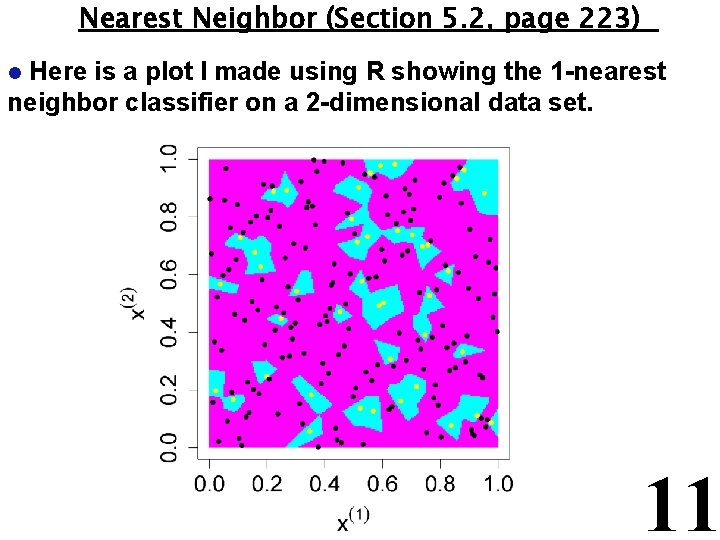Nearest Neighbor (Section 5. 2, page 223) Here is a plot I made using R showing the 1 -nearest neighbor classifier on a 2 -dimensional data set. l 11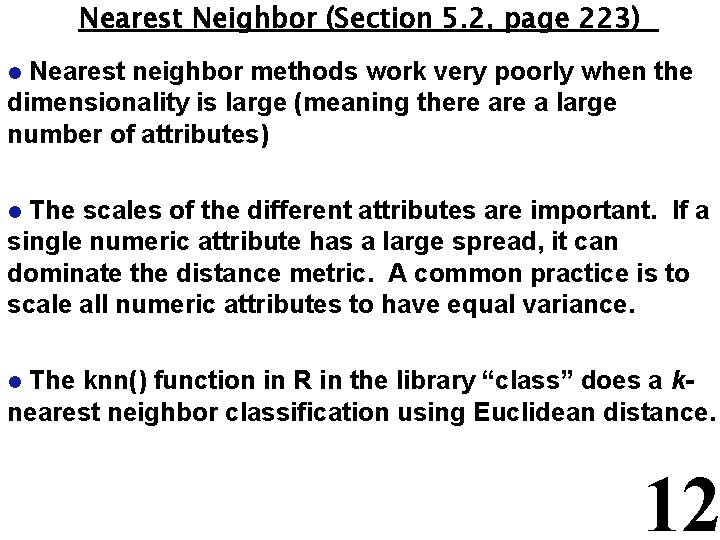Nearest Neighbor (Section 5. 2, page 223) Nearest neighbor methods work very poorly when the dimensionality is large (meaning there a large number of attributes) l The scales of the different attributes are important. If a single numeric attribute has a large spread, it can dominate the distance metric. A common practice is to scale all numeric attributes to have equal variance. l The knn() function in R in the library “class” does a knearest neighbor classification using Euclidean distance. l 12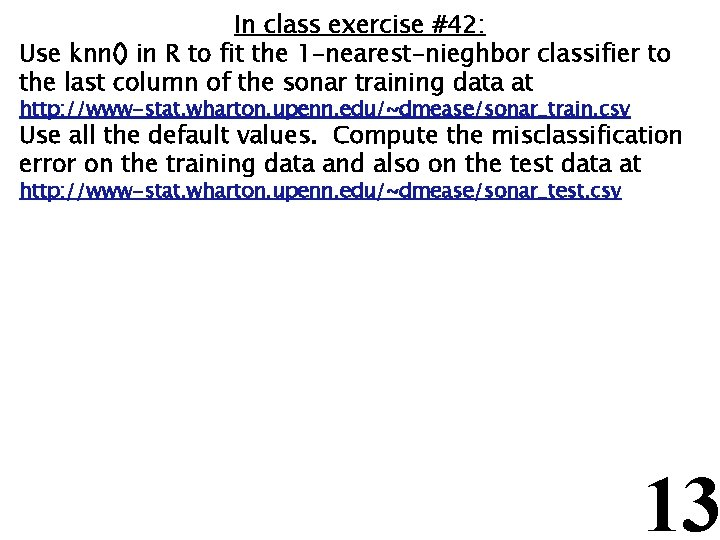In class exercise #42: Use knn() in R to fit the 1 -nearest-nieghbor classifier to the last column of the sonar training data at http: //www-stat. wharton. upenn. edu/~dmease/sonar_train. csv Use all the default values. Compute the misclassification error on the training data and also on the test data at http: //www-stat. wharton. upenn. edu/~dmease/sonar_test. csv 13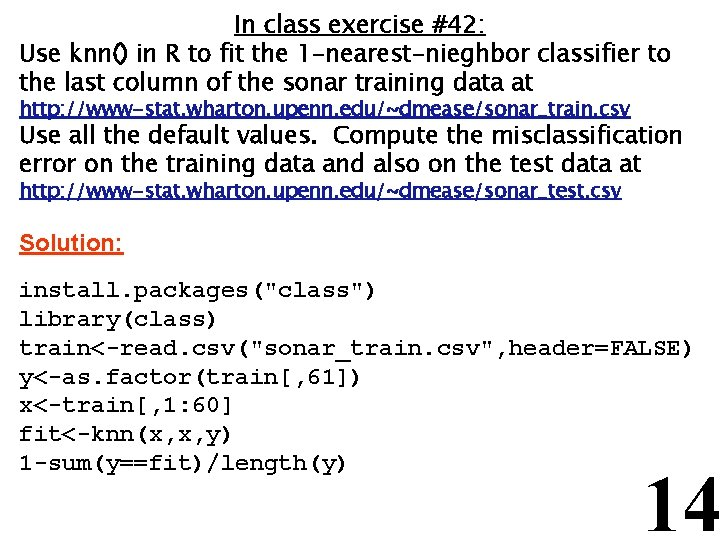In class exercise #42: Use knn() in R to fit the 1 -nearest-nieghbor classifier to the last column of the sonar training data at http: //www-stat. wharton. upenn. edu/~dmease/sonar_train. csv Use all the default values. Compute the misclassification error on the training data and also on the test data at http: //www-stat. wharton. upenn. edu/~dmease/sonar_test. csv Solution: install. packages("class") library(class) train<-read. csv("sonar_train. csv", header=FALSE) y<-as. factor(train[, 61]) x<-train[, 1: 60] fit<-knn(x, x, y) 1 -sum(y==fit)/length(y) 14In class exercise #42: Use knn() in R to fit the 1 -nearest-nieghbor classifier to the last column of the sonar training data at http: //www-stat. wharton. upenn. edu/~dmease/sonar_train. csv Use all the default values. Compute the misclassification error on the training data and also on the test data at http: //www-stat. wharton. upenn. edu/~dmease/sonar_test. csv Solution (continued): test<-read. csv("sonar_test. csv", header=FALSE) y_test<-as. factor(test[, 61]) x_test<-test[, 1: 60] fit_test<-knn(x, x_test, y) 1 -sum(y_test==fit_test)/length(y_test) 15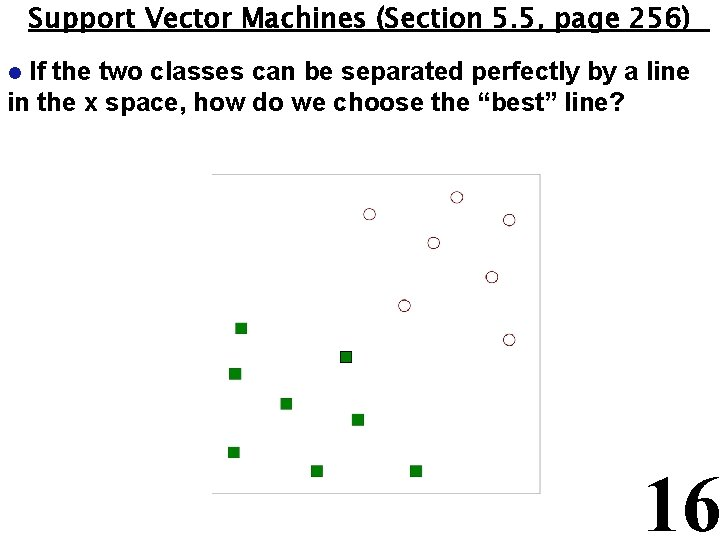Support Vector Machines (Section 5. 5, page 256) If the two classes can be separated perfectly by a line in the x space, how do we choose the “best” line? l 16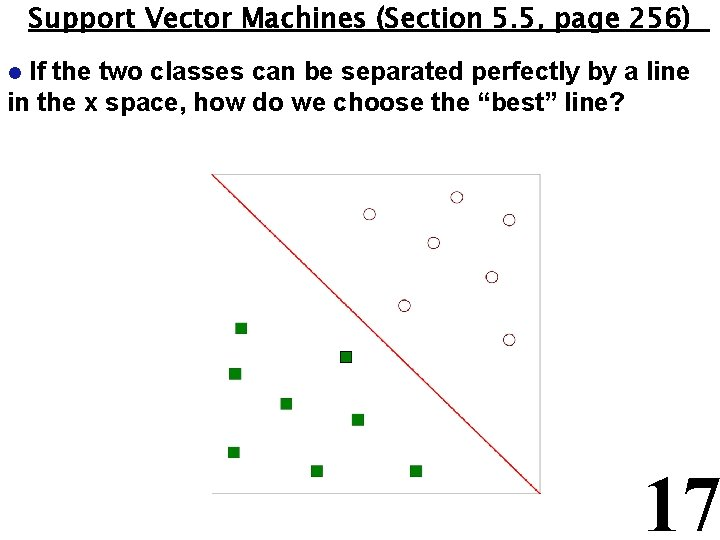Support Vector Machines (Section 5. 5, page 256) If the two classes can be separated perfectly by a line in the x space, how do we choose the “best” line? l 17Support Vector Machines (Section 5. 5, page 256) If the two classes can be separated perfectly by a line in the x space, how do we choose the “best” line? l 18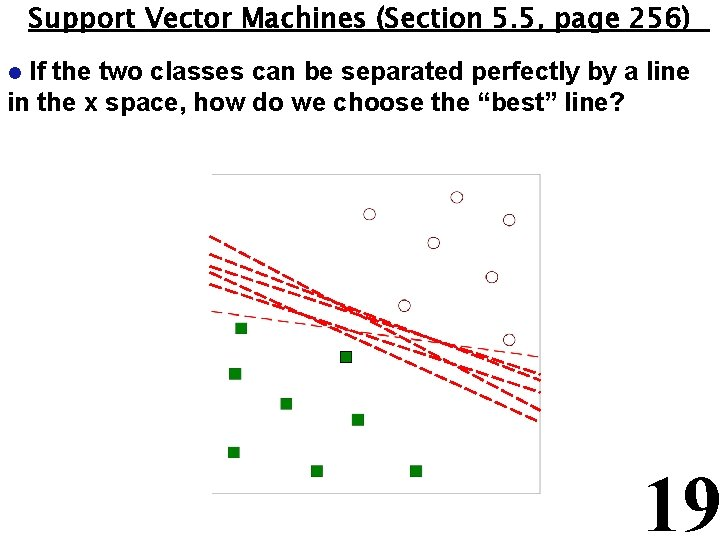Support Vector Machines (Section 5. 5, page 256) If the two classes can be separated perfectly by a line in the x space, how do we choose the “best” line? l 19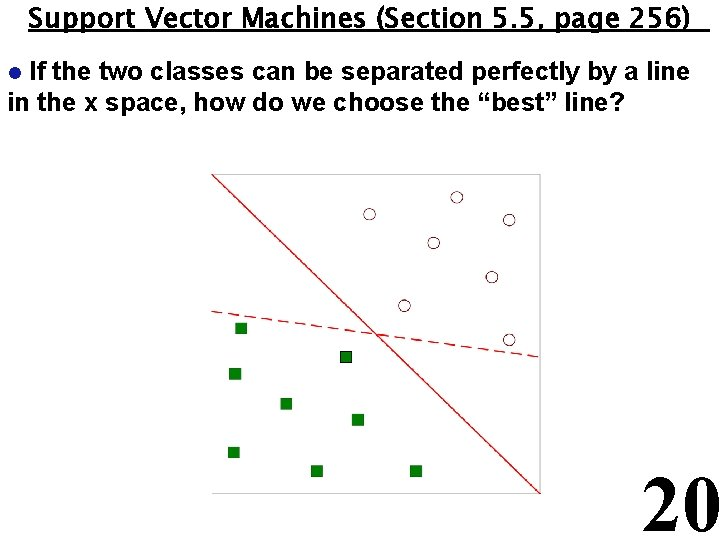Support Vector Machines (Section 5. 5, page 256) If the two classes can be separated perfectly by a line in the x space, how do we choose the “best” line? l 20Support Vector Machines (Section 5. 5, page 256) One solution is to choose the line (hyperplane) with the largest margin. The margin is the distance between the two parallel lines on either side. l B 1 B 2 b 21 b 22 margin b 12 b 11 21Support Vector Machines (Section 5. 5, page 256) l Here is the notation your book uses: 22Support Vector Machines (Section 5. 5, page 256) This can be formulated as a constrained optimization problem. l l We want to maximize l This is equivalent to minimizing l We have the following constraints So we have a quadratic objective function with linear constraints which means it is a convex optimization problem and we can use Lagrange multipliers l 23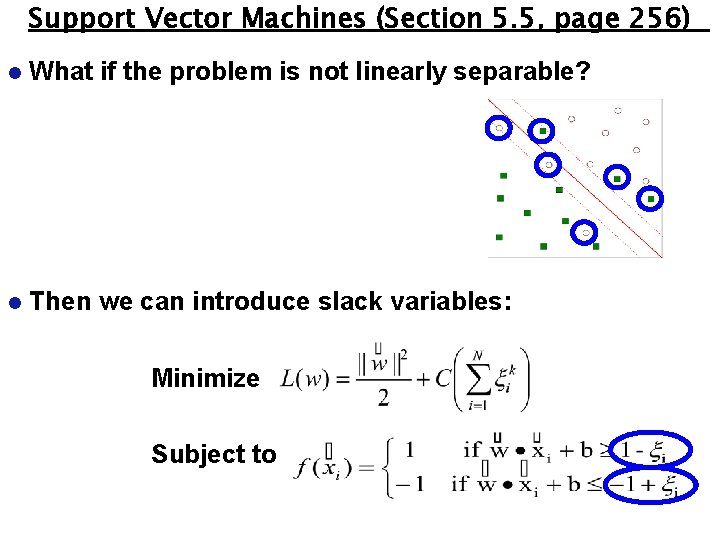Support Vector Machines (Section 5. 5, page 256) l What if the problem is not linearly separable? l Then we can introduce slack variables: Minimize Subject to 24Support Vector Machines (Section 5. 5, page 256) l What if the boundary is not linear? Then we can use transformations of the variables to map into a higher dimensional space l 25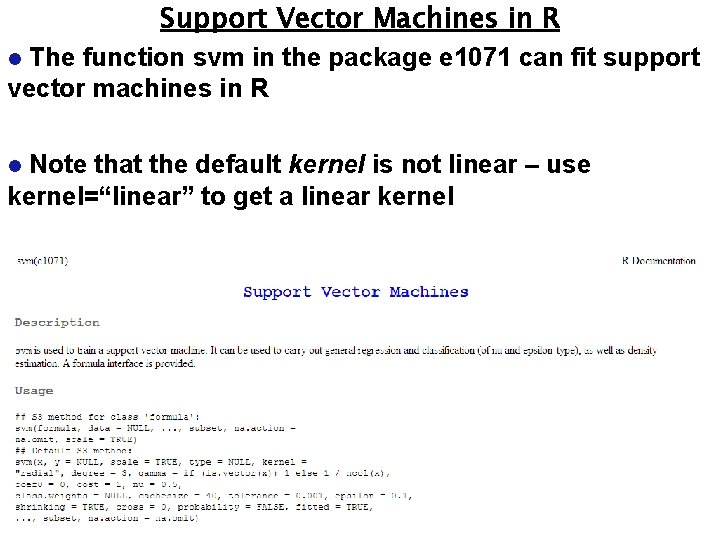Support Vector Machines in R The function svm in the package e 1071 can fit support vector machines in R l Note that the default kernel is not linear – use kernel=“linear” to get a linear kernel l 26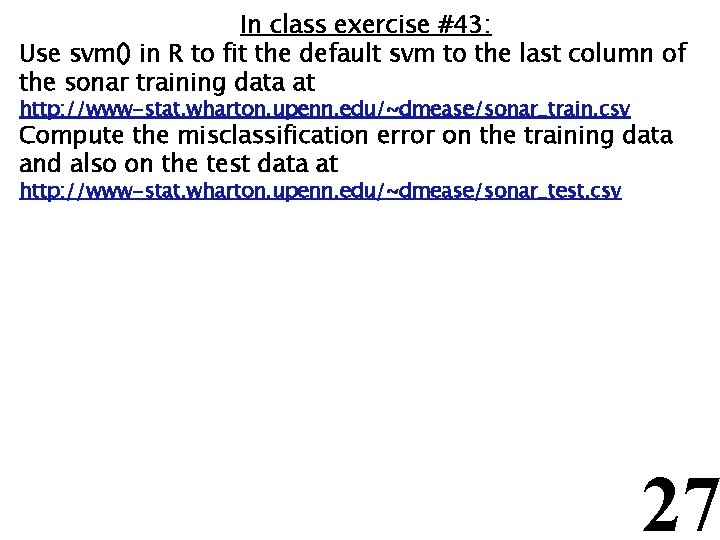In class exercise #43: Use svm() in R to fit the default svm to the last column of the sonar training data at http: //www-stat. wharton. upenn. edu/~dmease/sonar_train. csv Compute the misclassification error on the training data and also on the test data at http: //www-stat. wharton. upenn. edu/~dmease/sonar_test. csv 27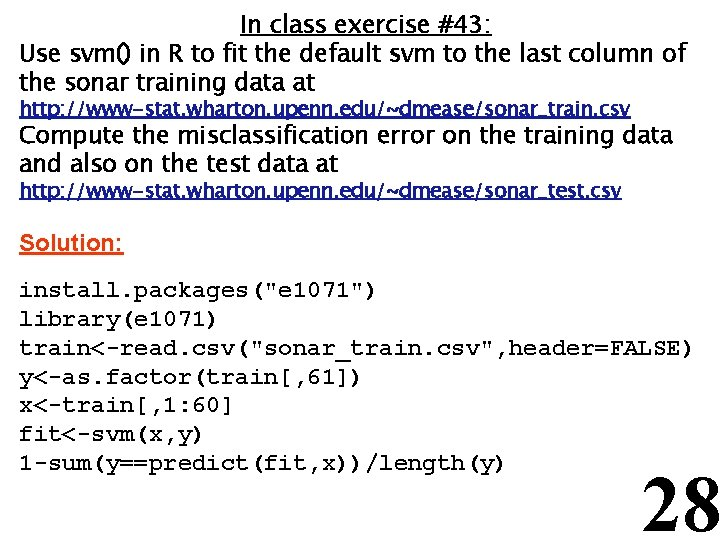In class exercise #43: Use svm() in R to fit the default svm to the last column of the sonar training data at http: //www-stat. wharton. upenn. edu/~dmease/sonar_train. csv Compute the misclassification error on the training data and also on the test data at http: //www-stat. wharton. upenn. edu/~dmease/sonar_test. csv Solution: install. packages("e 1071") library(e 1071) train<-read. csv("sonar_train. csv", header=FALSE) y<-as. factor(train[, 61]) x<-train[, 1: 60] fit<-svm(x, y) 1 -sum(y==predict(fit, x))/length(y) 28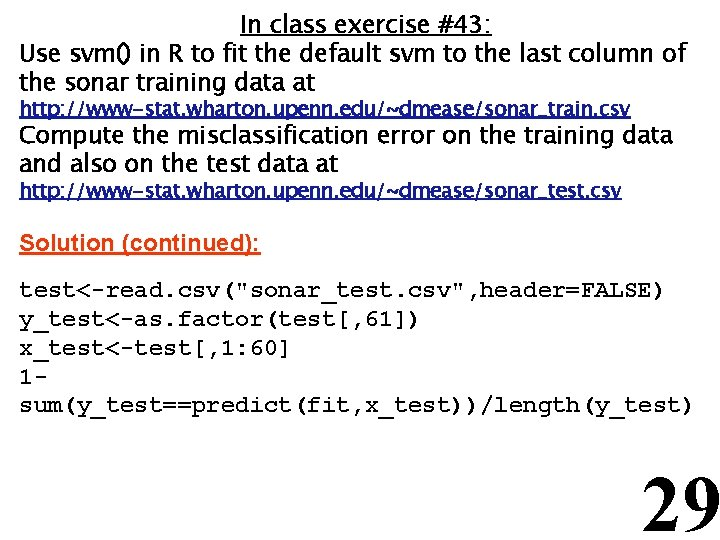In class exercise #43: Use svm() in R to fit the default svm to the last column of the sonar training data at http: //www-stat. wharton. upenn. edu/~dmease/sonar_train. csv Compute the misclassification error on the training data and also on the test data at http: //www-stat. wharton. upenn. edu/~dmease/sonar_test. csv Solution (continued): test<-read. csv("sonar_test. csv", header=FALSE) y_test<-as. factor(test[, 61]) x_test<-test[, 1: 60] 1 sum(y_test==predict(fit, x_test))/length(y_test) 29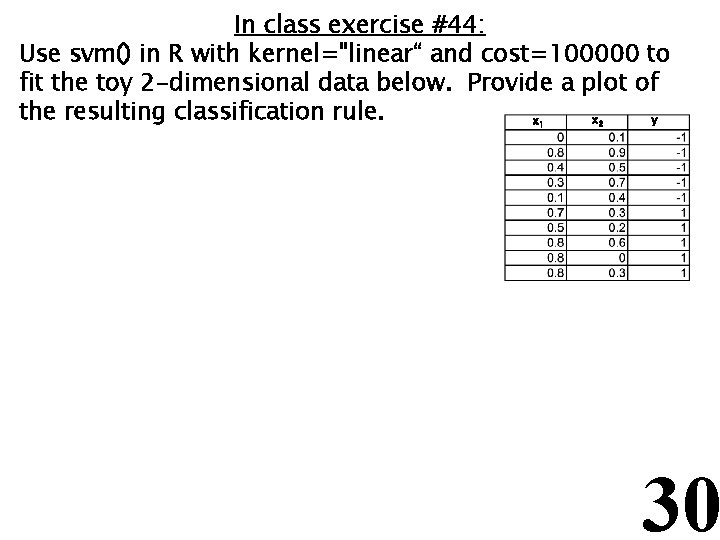In class exercise #44: Use svm() in R with kernel="linear“ and cost=100000 to fit the toy 2 -dimensional data below. Provide a plot of the resulting classification rule. x y x 1 2 30In class exercise #44: Use svm() in R with kernel="linear“ and cost=100000 to fit the toy 2 -dimensional data below. Provide a plot of the resulting classification rule. x y x 1 2 Solution: x<-matrix(c(0, . 1, . 8, . 9, . 4, . 5, . 3, . 7, . 1, . 4, . 7, . 3, . 5, . 2, . 8, . 6, . 8, 0, . 8, . 3), ncol=2, byrow=T) y<-as. factor(c(rep(-1, 5), rep(1, 5))) plot(x, pch=19, xlim=c(0, 1), ylim=c(0, 1), col=2*as. numeric(y), cex=2, xlab=expression(x), ylab=expression(x)) 31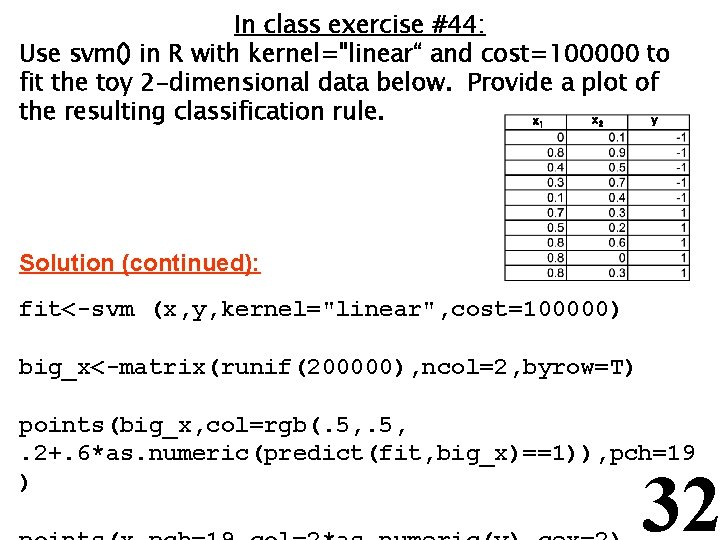In class exercise #44: Use svm() in R with kernel="linear“ and cost=100000 to fit the toy 2 -dimensional data below. Provide a plot of the resulting classification rule. x y x 1 2 Solution (continued): fit<-svm (x, y, kernel="linear", cost=100000) big_x<-matrix(runif(200000), ncol=2, byrow=T) points(big_x, col=rgb(. 5, . 2+. 6*as. numeric(predict(fit, big_x)==1)), pch=19 ) 32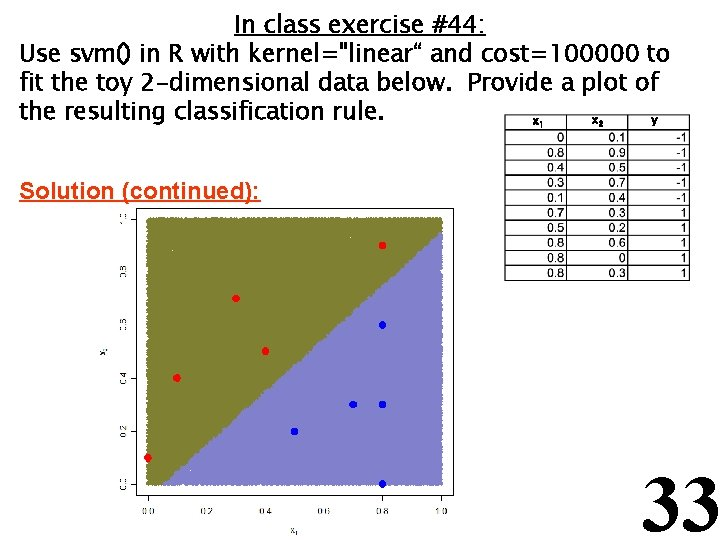In class exercise #44: Use svm() in R with kernel="linear“ and cost=100000 to fit the toy 2 -dimensional data below. Provide a plot of the resulting classification rule. x y x 1 2 Solution (continued): 33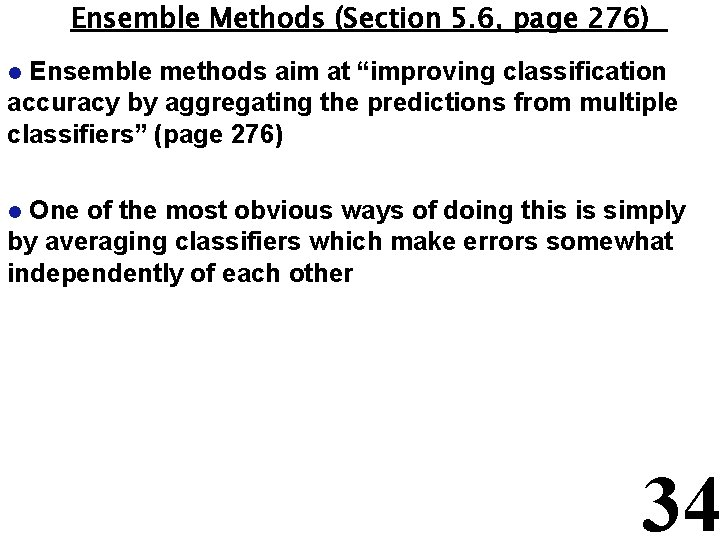Ensemble Methods (Section 5. 6, page 276) Ensemble methods aim at “improving classification accuracy by aggregating the predictions from multiple classifiers” (page 276) l One of the most obvious ways of doing this is simply by averaging classifiers which make errors somewhat independently of each other l 34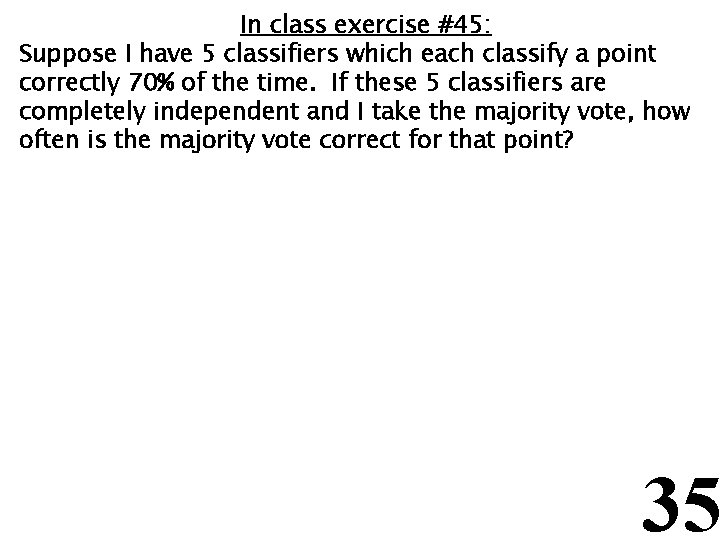In class exercise #45: Suppose I have 5 classifiers which each classify a point correctly 70% of the time. If these 5 classifiers are completely independent and I take the majority vote, how often is the majority vote correct for that point? 35In class exercise #45: Suppose I have 5 classifiers which each classify a point correctly 70% of the time. If these 5 classifiers are completely independent and I take the majority vote, how often is the majority vote correct for that point? Solution (continued): 10*. 7^3*. 3^2 + 5*. 7^4*. 3^1 +. 7^5 or 1 -pbinom(2, 5, . 7) 36In class exercise #46: Suppose I have 101 classifiers which each classify a point correctly 70% of the time. If these 101 classifiers are completely independent and I take the majority vote, how often is the majority vote correct for that point? 37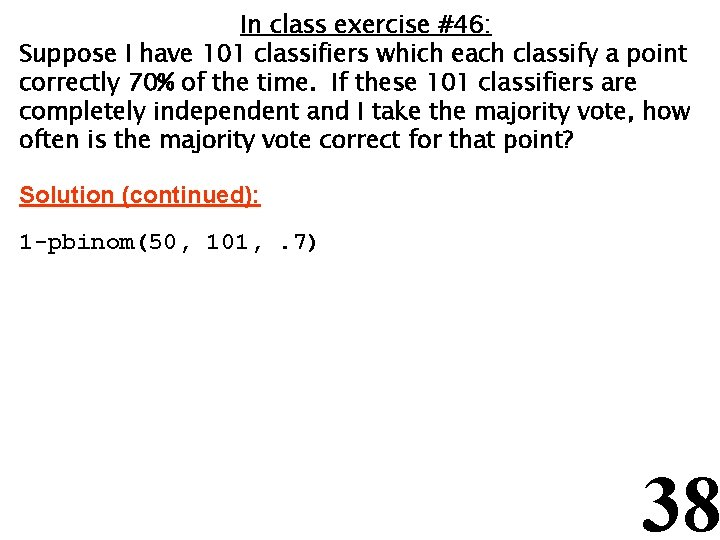In class exercise #46: Suppose I have 101 classifiers which each classify a point correctly 70% of the time. If these 101 classifiers are completely independent and I take the majority vote, how often is the majority vote correct for that point? Solution (continued): 1 -pbinom(50, 101, . 7) 38Ensemble Methods (Section 5. 6, page 276) Ensemble methods include -Bagging (page 283) -Random Forests (page 290) -Boosting (page 285) l Bagging builds different classifiers by training on repeated samples (with replacement) from the data l Random Forests averages many trees which are constructed with some amount of randomness l 39 Boosting combines simple base classifiers by upweighting data points which are classified incorrectly lRandom Forests (Section 5. 6. 6, page 290) One way to create random forests is to grow decision trees top down but at each terminal node consider only a random subset of attributes for splitting instead of all the attributes l l Random Forests are a very effective technique l They are based on the paper L. Breiman. Random forests. Machine Learning, 45: 5 -32, 2001 They can be fit in R using the function random. Forest() in the library random. Forest l 40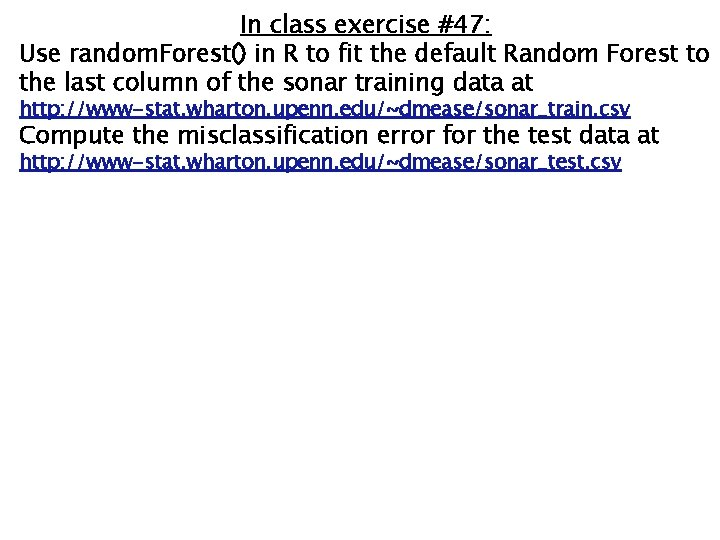In class exercise #47: Use random. Forest() in R to fit the default Random Forest to the last column of the sonar training data at http: //www-stat. wharton. upenn. edu/~dmease/sonar_train. csv Compute the misclassification error for the test data at http: //www-stat. wharton. upenn. edu/~dmease/sonar_test. csv 41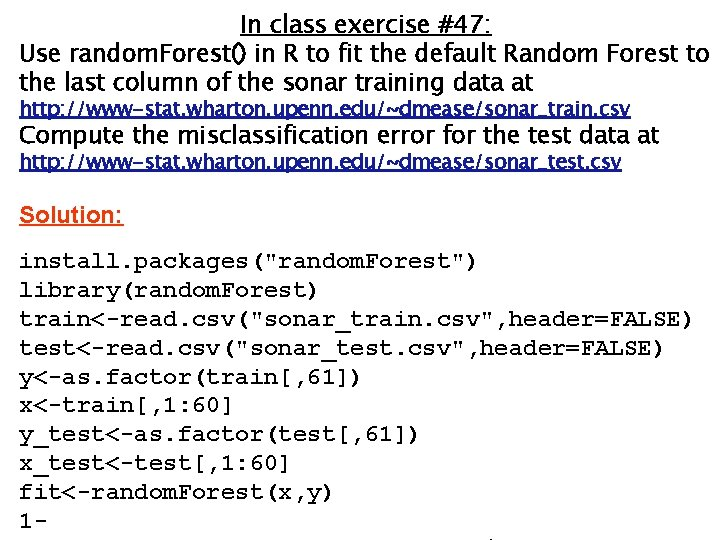In class exercise #47: Use random. Forest() in R to fit the default Random Forest to the last column of the sonar training data at http: //www-stat. wharton. upenn. edu/~dmease/sonar_train. csv Compute the misclassification error for the test data at http: //www-stat. wharton. upenn. edu/~dmease/sonar_test. csv Solution: install. packages("random. Forest") library(random. Forest) train<-read. csv("sonar_train. csv", header=FALSE) test<-read. csv("sonar_test. csv", header=FALSE) y<-as. factor(train[, 61]) x<-train[, 1: 60] y_test<-as. factor(test[, 61]) x_test<-test[, 1: 60] fit<-random. Forest(x, y) 1 - 42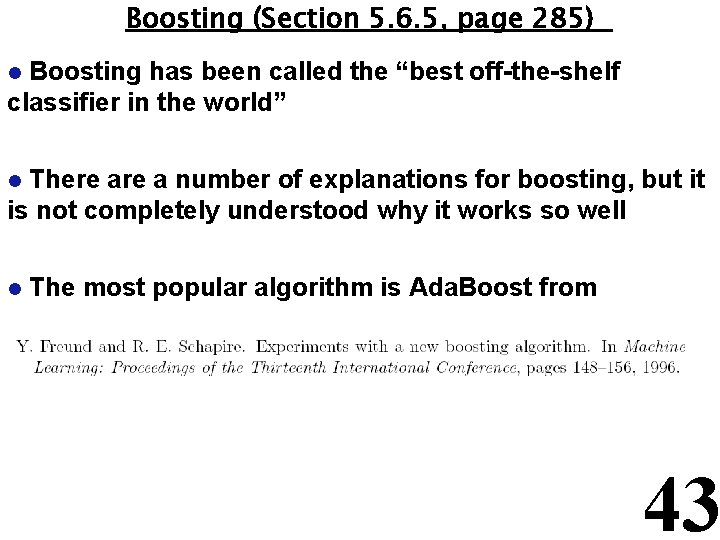Boosting (Section 5. 6. 5, page 285) Boosting has been called the “best off-the-shelf classifier in the world” l There a number of explanations for boosting, but it is not completely understood why it works so well l l The most popular algorithm is Ada. Boost from 43Boosting (Section 5. 6. 5, page 285) Boosting can use any classifier as its weak learner (base classifier) but decision trees are by far the most popular l Boosting usually gives zero training error, but rarely overfits which is very curious l 44Boosting (Section 5. 6. 5, page 285) Boosting works by upweighing points at each iteration which are misclassified l On paper, boosting looks like an optimization (similar to maximum likelihood estimation), but in practice it seems to benefit a lot from averaging like Random Forests does l There exist R libraries for boosting, but these are written by statisticians who have their own views of boosting, so I would not encourage you to use them l The best thing to do is to write code yourself since the algorithms are very basic l 45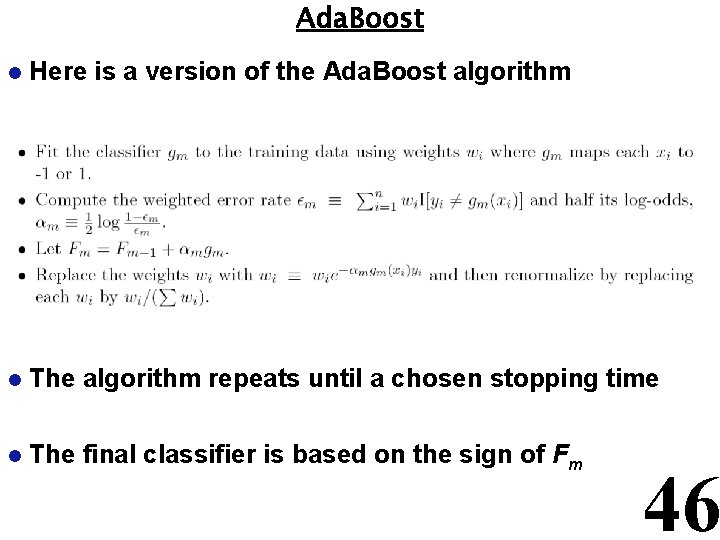Ada. Boost l Here is a version of the Ada. Boost algorithm l The algorithm repeats until a chosen stopping time l The final classifier is based on the sign of Fm 46In class exercise #48: Use R to fit the Ada. Boost classifier to the last column of the sonar training data at http: //www-stat. wharton. upenn. edu/~dmease/sonar_train. csv Plot the misclassification error for the training data and the test data at http: //www-stat. wharton. upenn. edu/~dmease/sonar_test. csv as a function of the iterations. Run the algorithm for 500 iterations. Use default rpart() as the base learner. Solution: train<-read. csv("sonar_train. csv", header=FALSE) test<-read. csv("sonar_test. csv", header=FALSE) y<-train[, 61] x<-train[, 1: 60] y_test<-test[, 61] x_test<-test[, 1: 60] 47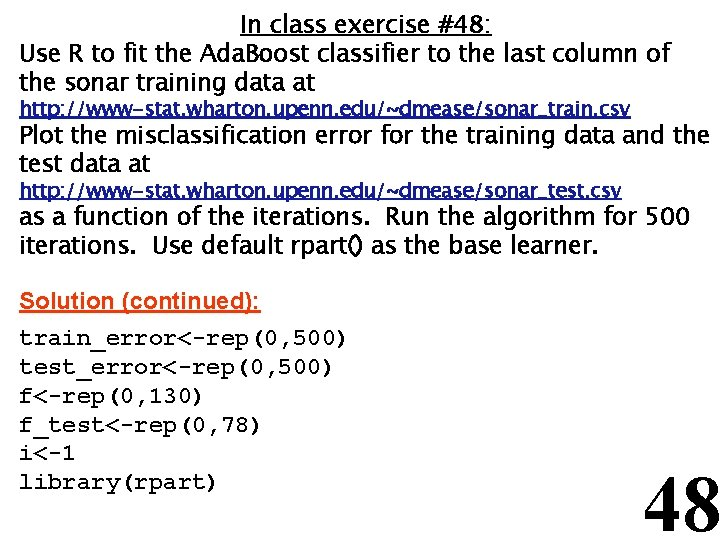In class exercise #48: Use R to fit the Ada. Boost classifier to the last column of the sonar training data at http: //www-stat. wharton. upenn. edu/~dmease/sonar_train. csv Plot the misclassification error for the training data and the test data at http: //www-stat. wharton. upenn. edu/~dmease/sonar_test. csv as a function of the iterations. Run the algorithm for 500 iterations. Use default rpart() as the base learner. Solution (continued): train_error<-rep(0, 500) test_error<-rep(0, 500) f<-rep(0, 130) f_test<-rep(0, 78) i<-1 library(rpart) 48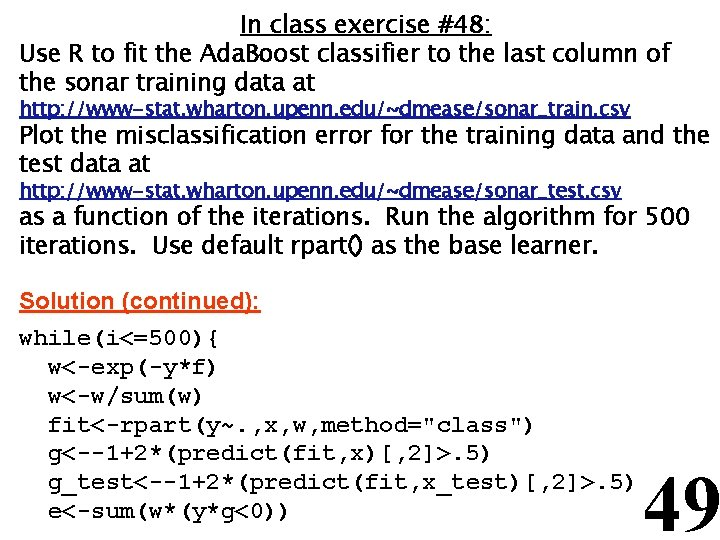In class exercise #48: Use R to fit the Ada. Boost classifier to the last column of the sonar training data at http: //www-stat. wharton. upenn. edu/~dmease/sonar_train. csv Plot the misclassification error for the training data and the test data at http: //www-stat. wharton. upenn. edu/~dmease/sonar_test. csv as a function of the iterations. Run the algorithm for 500 iterations. Use default rpart() as the base learner. Solution (continued): while(i<=500){ w<-exp(-y*f) w<-w/sum(w) fit<-rpart(y~. , x, w, method="class") g<--1+2*(predict(fit, x)[, 2]>. 5) g_test<--1+2*(predict(fit, x_test)[, 2]>. 5) e<-sum(w*(y*g<0)) 49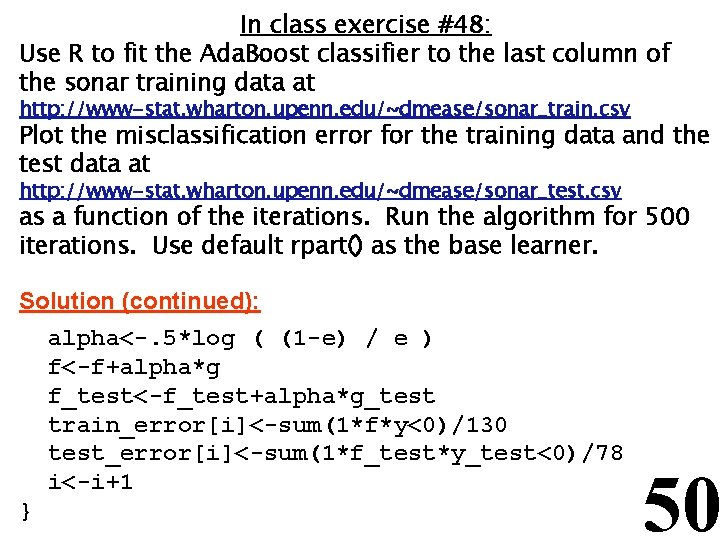In class exercise #48: Use R to fit the Ada. Boost classifier to the last column of the sonar training data at http: //www-stat. wharton. upenn. edu/~dmease/sonar_train. csv Plot the misclassification error for the training data and the test data at http: //www-stat. wharton. upenn. edu/~dmease/sonar_test. csv as a function of the iterations. Run the algorithm for 500 iterations. Use default rpart() as the base learner. Solution (continued): alpha<-. 5*log ( (1 -e) / e ) f<-f+alpha*g f_test<-f_test+alpha*g_test train_error[i]<-sum(1*f*y<0)/130 test_error[i]<-sum(1*f_test*y_test<0)/78 i<-i+1 } 50In class exercise #48: Use R to fit the Ada. Boost classifier to the last column of the sonar training data at http: //www-stat. wharton. upenn. edu/~dmease/sonar_train. csv Plot the misclassification error for the training data and the test data at http: //www-stat. wharton. upenn. edu/~dmease/sonar_test. csv as a function of the iterations. Run the algorithm for 500 iterations. Use default rpart() as the base learner. Solution (continued): plot(seq(1, 500), test_error, type="l", ylim=c(0, . 5), ylab="Error Rate", xlab="Iterations", lwd=2) lines(train_error, lwd=2, col="purple") legend(4, . 5, c("Training Error", "Test Error"), col=c("purple", "black"), lwd=2) 51In class exercise #48: Use R to fit the Ada. Boost classifier to the last column of the sonar training data at http: //www-stat. wharton. upenn. edu/~dmease/sonar_train. csv Plot the misclassification error for the training data and the test data at http: //www-stat. wharton. upenn. edu/~dmease/sonar_test. csv as a function of the iterations. Run the algorithm for 500 iterations. Use default rpart() as the base learner. Solution (continued): 52